Function Repository Resource:

# MakePetriNet

Make an abstract representation of a Petri net configuration

Contributed by: Jonathan Gorard
 ResourceFunction["MakePetriNet"][p,t,a,init] makes a Petri net using the list of places p, the list of transitions t and the list of arcs a, with initial token distribution init. ResourceFunction["MakePetriNet"][assoc,init] makes a Petri net using the association of places, transitions and arcs assoc, with initial token distribution init.

## Details

A Petri net is an abstract mathematical representation of a distributed system (frequently used for describing chemical reactions, biological processes, concurrent computing, etc.) consisting of a directed bipartite graph whose vertices are classified as either "places" or "transitions". The directed edges connecting places and tokens together are known as "arcs".
Each place can contain a number of "tokens", and a given transition will "fire" if the places connected to it by input arcs all contain at least one token. When a transition fires, it redistributes tokens from places connected to it by input arcs to places connected to it by output arcs.
ResourceFunction["MakePetriNet"] supports the specification of Petri nets either by three lists (places p, transitions t and arcs a), or by an explicit association of the form <|"Places"p,"Transitions"t,"Arcs"a|>. The list of arcs a should be a list of either rules or DirectedEdge objects (each connecting a place to a transition, or vice versa).
If ResourceFunction["MakePetriNet"] succeeds in constructing the specified Petri net, then it returns a PetriNetObject expression.
The list init should be a list of integers specifying how many initial tokens to assign to each place. If a list init is not given, ResourceFunction["MakePetriNet"] will assume that each place contains no tokens.
In PetriNetObject, the following properties are supported:
 "AssociationForm" Petri net represented as an association of places, transitions and arcs "Places" list of places in the Petri net "PlaceCount" number of places in the Petri net "Transitions" list of transitions in the Petri net "TransitionCount" number of transitions in the Petri net "Arcs" list of arcs in the Petri net "ArcCount" number of arcs in the Petri net "Tokens" list of token numbers associated to each place in the Petri net "TokenCount" total number of tokens across all places in the Petri net "UnlabeledGraph" directed graph form of the Petri net without token counts represented graphically "LabeledGraph" directed graph form of the Petri net with token counts represented graphically "WeightedGraph" directed graph form of the Petri net with token counts represented as vertex weights
By convention, ResourceFunction["MakePetriNet"] renders places as circles, transitions as squares, arcs as directed edges and tokens as black dots.

## Examples

### Basic Examples (3)

Construct a simple Petri net from lists of four places, three transitions and eight arcs, with an initial distribution of six tokens:

 In:=Out=Show the unlabeled graph:

 In:=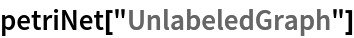Out=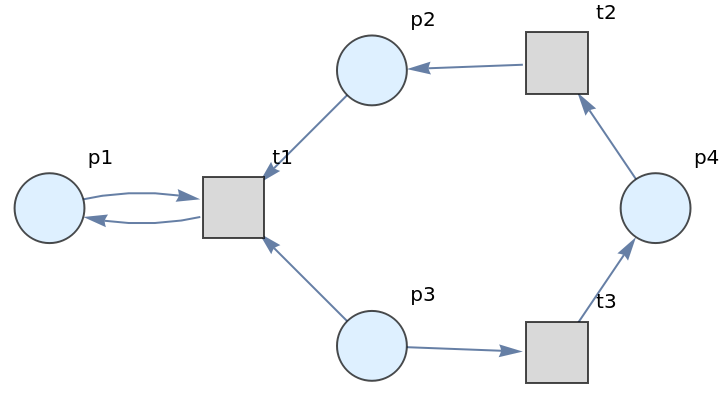Show the labeled graph with token counts represented graphically:

 In:=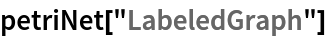Out=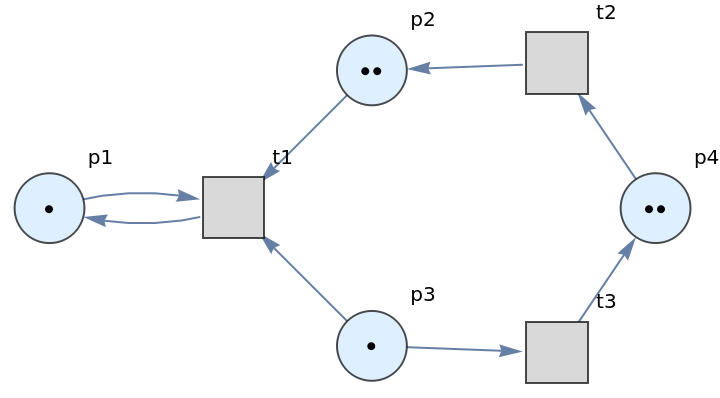Show the weighted graph with token counts represented as vertex weights:

 In:=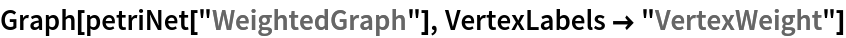Out=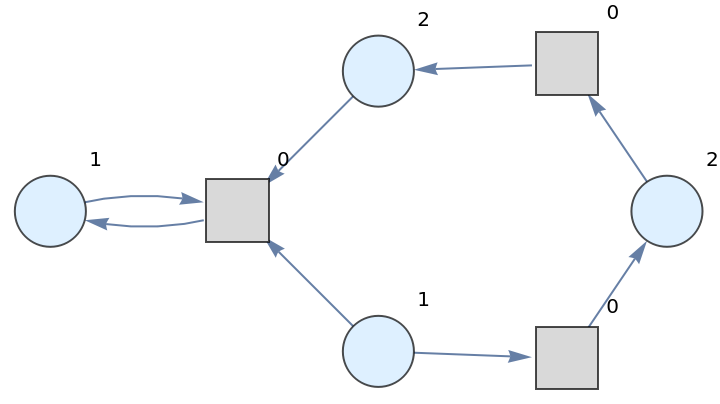Construct a Petri net representing a simple reversible chemical reaction from an association of three places, two transitions and six arcs, with an initial distribution of twelve tokens:

 In:=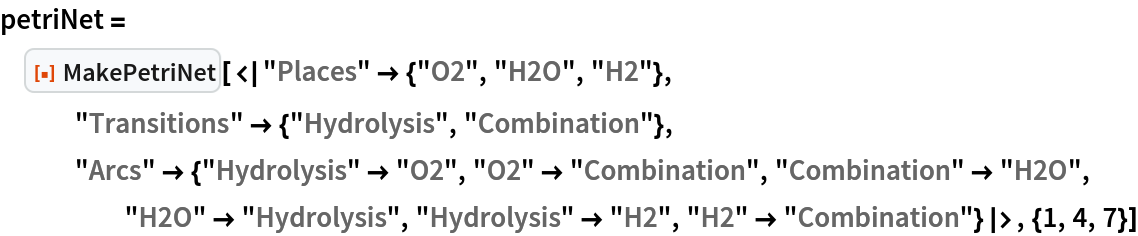Out=Show the labeled graph with token counts represented graphically:

 In:=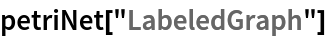Out=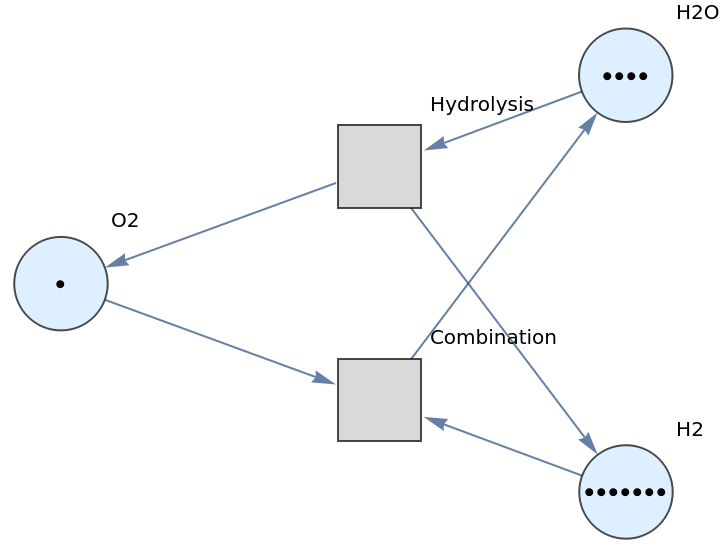Show the association of places, transitions and arcs:

 In:=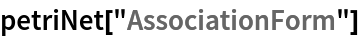Out=Show the list of arcs (directed edges):

 In:=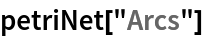Out=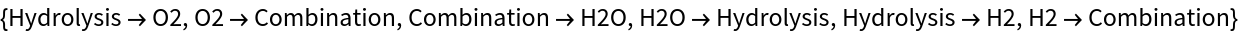Show the distribution of tokens across places:

 In:=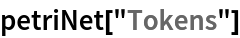Out=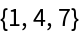Construct a more complicated Petri net representing a concurrent communications protocol between two agents:

 In:=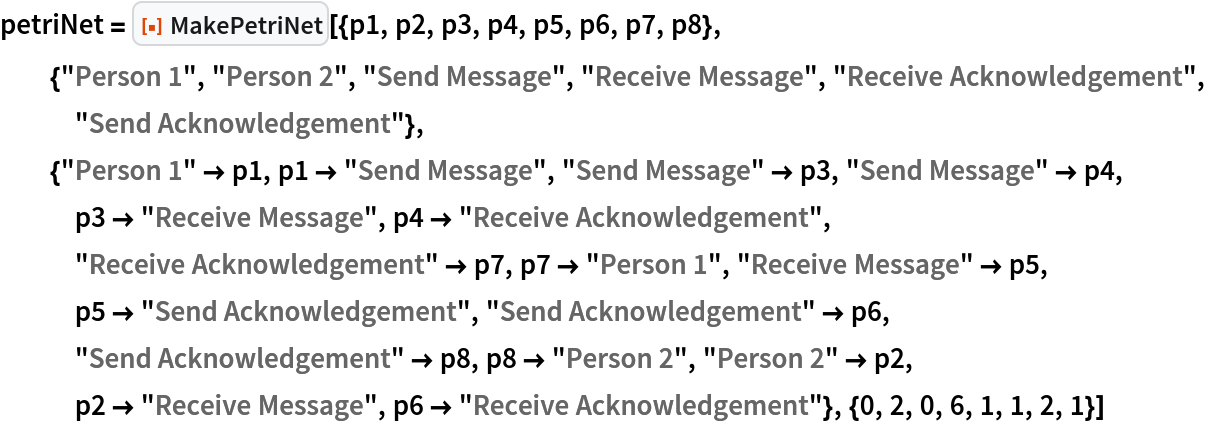Out=Show the labeled graph with token counts represented graphically:

 In:=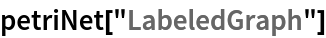Out=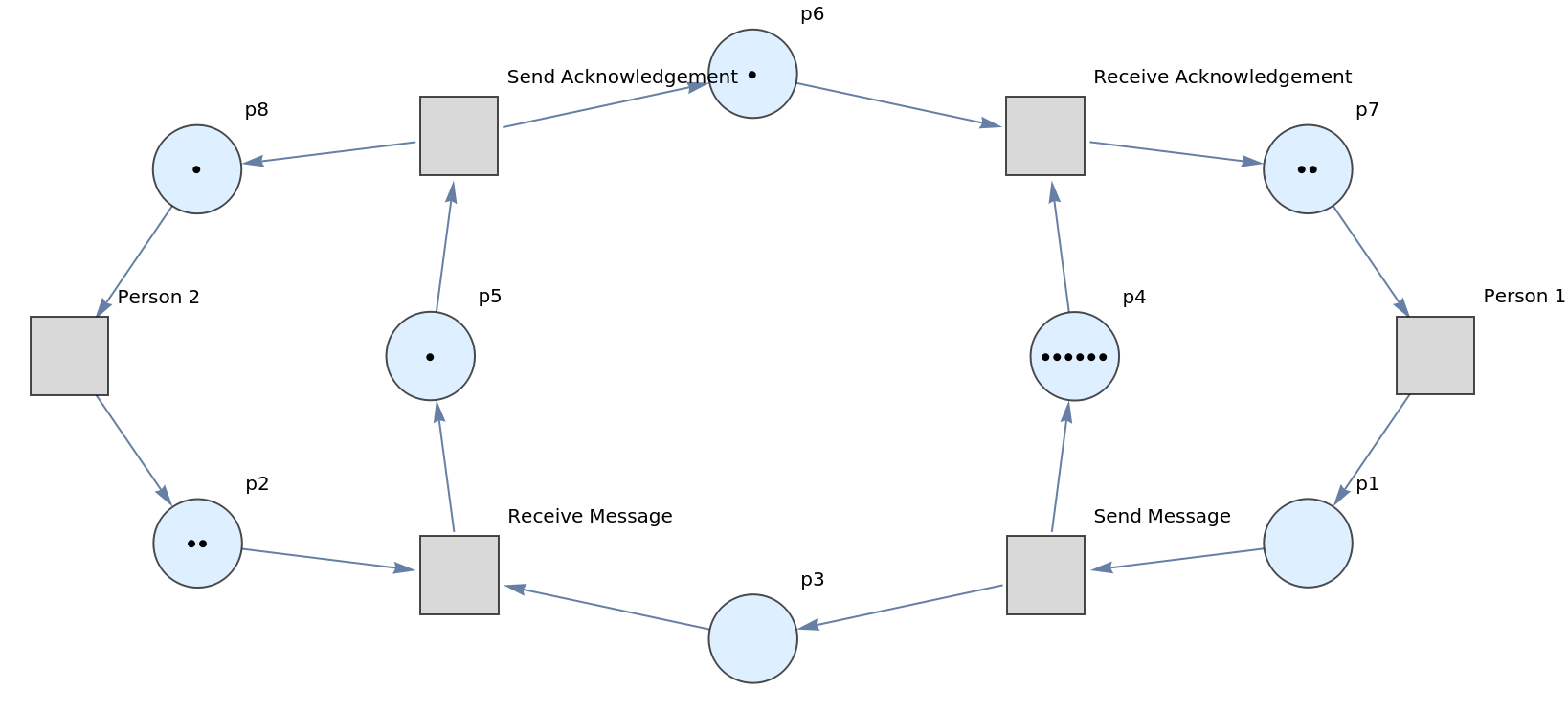Show the list of transitions:

 In:=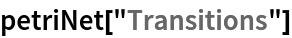Out=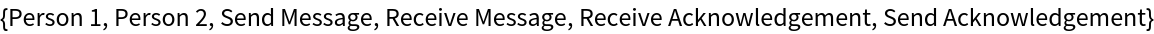### Scope (2)

If MakePetriNet is called without an explicit list of token numbers, it is assumed that each place contains no tokens:

 In:=Out=Show the labeled graph:

 In:=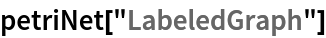Out=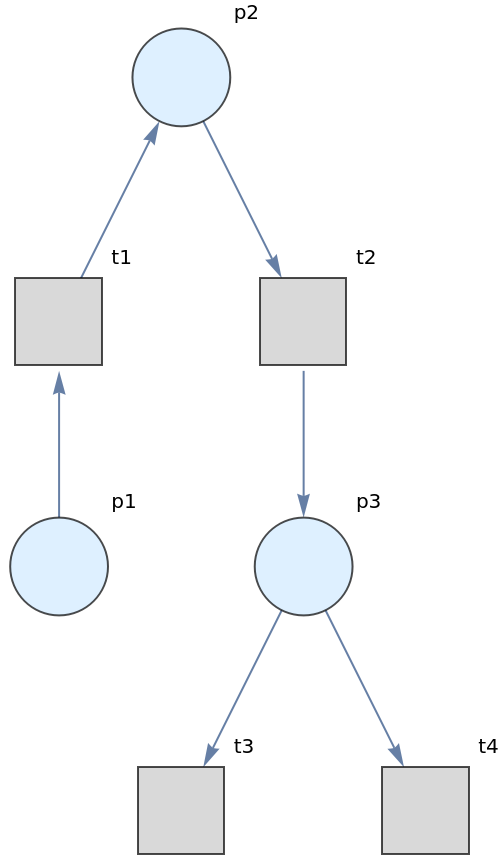Show the default distribution of tokens across places:

 In:=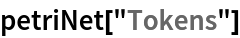Out=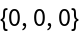Construct a Petri net representing a simple producer-consumer problem in concurrency theory:

 In:=Out=Show the list of properties:

 In:=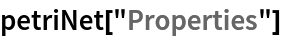Out=Show the representation of the Petri net as an association of places, transitions and arcs:

 In:=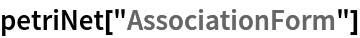Out=Show the list of places in the Petri net:

 In:=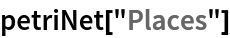Out=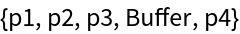Show the number of places in the Petri net:

 In:=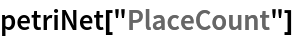Out=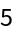Show the list of transitions in the Petri net:

 In:=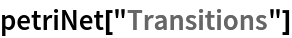Out=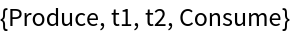Show the number of transitions in the Petri net:

 In:=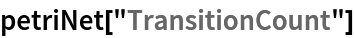Out=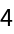Show the list of arcs in the Petri net:

 In:=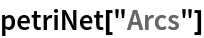Out=Show the number of arcs in the Petri net:

 In:=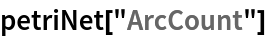Out=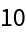Show the list of token numbers associated to each place in the Petri net:

 In:=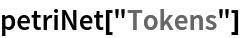Out=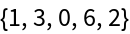Show the total number of tokens across all places in the Petri net:

 In:=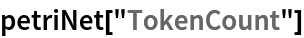Out=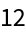Show the directed graph form of the Petri net without token counts represented graphically:

 In:=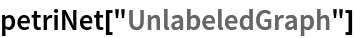Out=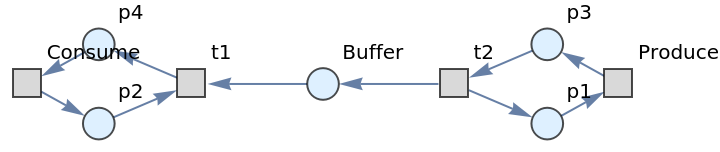Show the directed graph form of the Petri net with token counts represented graphically:

 In:=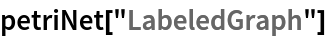Out=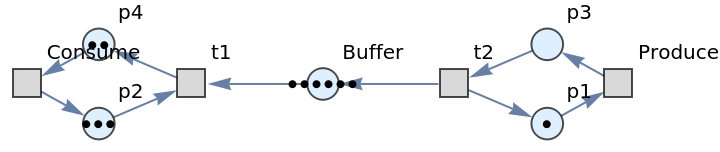Show the directed graph form of the Petri net with token counts represented as vertex weights:

 In:=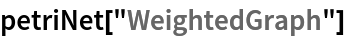Out=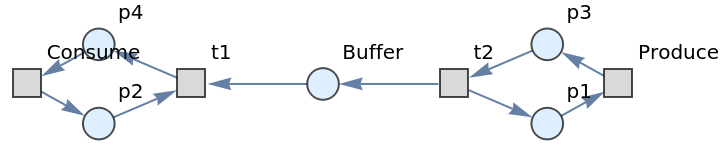In:=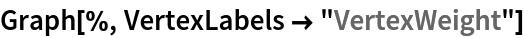Out=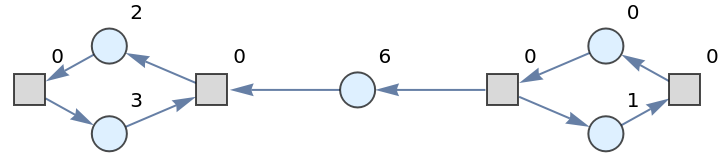Jonathan Gorard

## Version History

• 1.0.0 – 22 November 2021